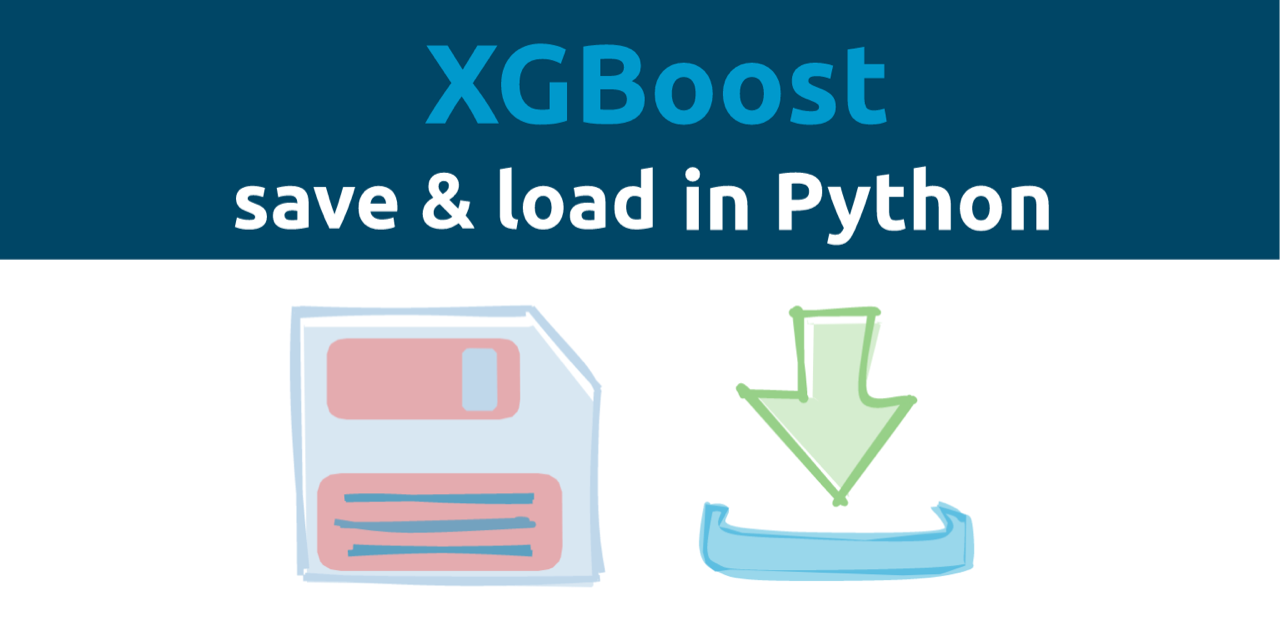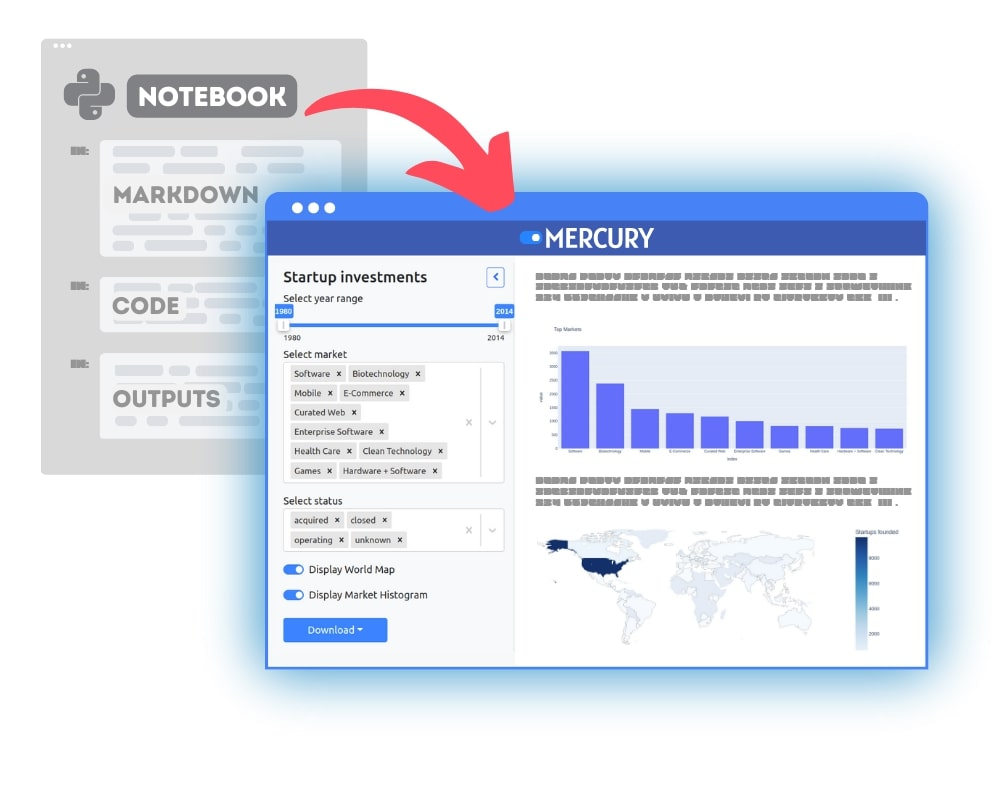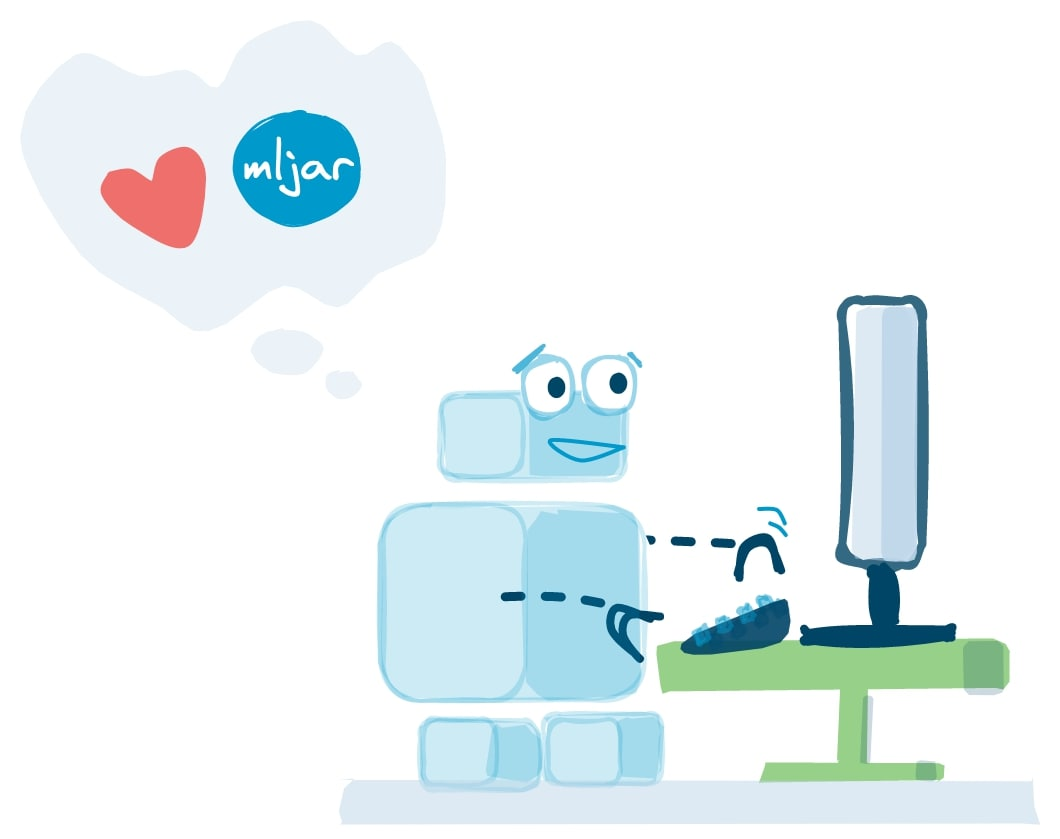Xgboost is a powerful gradient boosting framework. It provides interfaces in many languages: Python, R, Java, C++, Juila, Perl, and Scala. In this post, I will show you how to save and load Xgboost models in Python. The Xgboost provides several Python API types, that can be a source of confusion at the beginning of the Machine Learning journey. I will try to show different ways for saving and loading the Xgboost models, and show which one is the safest.

The Xgboost model can be trained in two ways:

• we can use python API that connects Python with Xgboost internals. It is called `Learning API` in the Xgboost documentation.
• or we can use Xgboost API that provides scikit-learn interface. The documentation of scikit-learn compatible API.

Depending on which way you use for training, the saving will be slightly different.

We are using Xgboost in version `2.0.0` in this article.

Let’s create the data:

``````import xgboost as xgb
from sklearn.datasets import make_classification
from sklearn.model_selection import train_test_split

print(xgb.__version__)
# I'm using Xgboost in version `2.0.0`.

# create example data
X, y = make_classification(n_samples=100,
n_informative=5,
n_classes=2)
X_train, X_test, y_train, y_test = train_test_split(X, y, test_size=0.25)
``````

## Xgboost Learning API

Let’s train Xgboost with learning API:

``````# We need to prepare data as DMatrix objects
train = xgb.DMatrix(X_train, y_train)
test = xgb.DMatrix(X_test, y_test)

# We need to define parameters as dict
params = {
"learning_rate": 0.01,
"max_depth": 3
}
# training, we set the early stopping rounds parameter
model_xgb = xgb.train(params,
train, evals=[(train, "train"), (test, "validation")],
num_boost_round=100, early_stopping_rounds=20)
``````

We will get the output like below:

``````	train-rmse:0.49644	validation-rmse:0.49860
	train-rmse:0.49232	validation-rmse:0.49773
	train-rmse:0.48917	validation-rmse:0.49637
	train-rmse:0.48535	validation-rmse:0.49523
	train-rmse:0.48226	validation-rmse:0.49371
	train-rmse:0.47921	validation-rmse:0.49222
	train-rmse:0.47584	validation-rmse:0.49100
	train-rmse:0.47284	validation-rmse:0.48959
	train-rmse:0.46894	validation-rmse:0.48889
	train-rmse:0.46530	validation-rmse:0.48793
	train-rmse:0.46239	validation-rmse:0.48640
	train-rmse:0.45893	validation-rmse:0.48558
	train-rmse:0.45516	validation-rmse:0.48499
	train-rmse:0.45233	validation-rmse:0.48374
	train-rmse:0.44918	validation-rmse:0.48272
	train-rmse:0.44550	validation-rmse:0.48220
	train-rmse:0.44275	validation-rmse:0.48120
	train-rmse:0.43935	validation-rmse:0.48045
	train-rmse:0.43666	validation-rmse:0.47911
	train-rmse:0.43343	validation-rmse:0.47847
	train-rmse:0.43011	validation-rmse:0.47780
	train-rmse:0.42749	validation-rmse:0.47674
	train-rmse:0.42434	validation-rmse:0.47616
	train-rmse:0.42111	validation-rmse:0.47556
	train-rmse:0.41856	validation-rmse:0.47449
	train-rmse:0.41516	validation-rmse:0.47418
	train-rmse:0.41213	validation-rmse:0.47368
	train-rmse:0.40900	validation-rmse:0.47316
	train-rmse:0.40654	validation-rmse:0.47218
	train-rmse:0.40359	validation-rmse:0.47173
	train-rmse:0.40033	validation-rmse:0.47154
	train-rmse:0.39763	validation-rmse:0.47090
	train-rmse:0.39526	validation-rmse:0.47020
	train-rmse:0.39208	validation-rmse:0.47007
	train-rmse:0.38925	validation-rmse:0.46972
	train-rmse:0.38632	validation-rmse:0.46936
	train-rmse:0.38404	validation-rmse:0.46873
	train-rmse:0.38128	validation-rmse:0.46843
	train-rmse:0.37843	validation-rmse:0.46814
	train-rmse:0.37572	validation-rmse:0.46788
	train-rmse:0.37352	validation-rmse:0.46710
	train-rmse:0.37054	validation-rmse:0.46709
	train-rmse:0.36810	validation-rmse:0.46656
	train-rmse:0.36595	validation-rmse:0.46578
	train-rmse:0.36304	validation-rmse:0.46580
	train-rmse:0.36047	validation-rmse:0.46551
	train-rmse:0.35780	validation-rmse:0.46532
	train-rmse:0.35573	validation-rmse:0.46480
	train-rmse:0.35322	validation-rmse:0.46465
	train-rmse:0.35042	validation-rmse:0.46474
	train-rmse:0.34816	validation-rmse:0.46432
	train-rmse:0.34616	validation-rmse:0.46384
	train-rmse:0.34343	validation-rmse:0.46396
	train-rmse:0.34103	validation-rmse:0.46376
	train-rmse:0.33909	validation-rmse:0.46311
	train-rmse:0.33661	validation-rmse:0.46304
	train-rmse:0.33427	validation-rmse:0.46322
	train-rmse:0.33183	validation-rmse:0.46316
	train-rmse:0.32995	validation-rmse:0.46283
	train-rmse:0.32737	validation-rmse:0.46301
	train-rmse:0.32511	validation-rmse:0.46311
	train-rmse:0.32275	validation-rmse:0.46309
	train-rmse:0.32094	validation-rmse:0.46252
	train-rmse:0.31844	validation-rmse:0.46274
	train-rmse:0.31625	validation-rmse:0.46298
	train-rmse:0.31397	validation-rmse:0.46301
	train-rmse:0.31183	validation-rmse:0.46326
	train-rmse:0.31010	validation-rmse:0.46273
	train-rmse:0.30770	validation-rmse:0.46298
	train-rmse:0.30549	validation-rmse:0.46304
	train-rmse:0.30342	validation-rmse:0.46319
	train-rmse:0.30175	validation-rmse:0.46289
	train-rmse:0.29943	validation-rmse:0.46317
	train-rmse:0.29730	validation-rmse:0.46326
	train-rmse:0.29529	validation-rmse:0.46355
	train-rmse:0.29368	validation-rmse:0.46328
	train-rmse:0.29143	validation-rmse:0.46358
	train-rmse:0.28937	validation-rmse:0.46370
	train-rmse:0.28743	validation-rmse:0.46389
	train-rmse:0.28589	validation-rmse:0.46346
	train-rmse:0.28371	validation-rmse:0.46379
	train-rmse:0.28172	validation-rmse:0.46392
	train-rmse:0.27984	validation-rmse:0.46424
``````

We can see that there are trained 83 trees. Let’s check the optimal tree number:

``````model_xgb.best_iteration

# result
> 63
``````

I will show you something that might surprise you. Let’s compute the predictions:

``````model_xgb.predict(test)

# result
> array([0.37061724, 0.23207052, 0.40625256, 0.28753477, 0.516009  ,
0.23207052, 0.5257586 , 0.3699053 , 0.35333863, 0.75463873,
0.2718957 , 0.74117696, 0.7306833 , 0.2913912 , 0.5032675 ,
0.35681653, 0.5884493 , 0.28920862, 0.516009  , 0.5212214 ,
0.23207052, 0.23207052, 0.7434248 , 0.23207052, 0.28405687],
dtype=float32)
``````

Again, let’s compute the predictions with an additional parameter ntree_limit:

``````model_xgb.predict(test, iteration_range=(0, model_xgb.best_iteration+1))

# result
> array([0.39995873, 0.2791416 , 0.4064596 , 0.32292357, 0.53171337,
0.2791416 , 0.52718186, 0.39682925, 0.38713488, 0.71079475,
0.29251572, 0.7001796 , 0.68683934, 0.3316707 , 0.5189719 ,
0.39087865, 0.5695737 , 0.33654556, 0.53171337, 0.5270995 ,
0.2791416 , 0.2791416 , 0.6995808 , 0.2791416 , 0.3191798 ],
dtype=float32)
``````

You see the difference in the predicted values! By default, the `predict()` method is not using an optimal number of trees. You need to specify the number of trees by yourself, by setting the iteration_range parameter.

### Save the Xgboost Booster object

There are two methods that can make the confusion:

• `save_model()`,
• `dump_model()`.

For saving and loading the model the `save_model()` should be used. The `dump_model()` is for model exporting which should be used for further model interpretation, for example visualization.

OK, so we will use `save_model()`. The next thing to remember is the extension of the saved file. If it will be `*.json` then the model will be saved in `json` format. Otherwise, it will be saved in text format.

Let’s check:

``````# save to JSON
model_xgb.save_model("model.json")
# save to text format
model_xgb.save_model("model.txt")
``````

There is a difference in file size. The `model.json` file has `100.8 KB` and the `model.txt` file has `57.9 KB` (much smaller).

``````model_xgb_2 = xgb.Booster()
``````

You can also load the model from the `model.txt` file. They will be the same. And now the surprise, let’s check the optimal number of trees:

``````model_xgb_2.best_iteration

# result
> ---------------------------------------------------------------------------
AttributeError                            Traceback (most recent call last)
<ipython-input-139-4b9915a166cd> in <module>
----> 1 model_xgb_2.best_iteration

AttributeError: 'Booster' object has no attribute 'best_iteration'

``````

That’s right the `best_iteration` variable is not saved. You must be very careful with this API. Let’s take a look at scikit-learn compatible API (it is much user friendly!).

## Xgboost with Scikit-learn API

Let’s train the Xgboost model with scikit-learn compatible API:

``````# training
model = xgb.XGBRegressor(n_estimators=100, max_depth=3, learning_rate=0.01,
early_stopping_rounds=20)
model.fit(X_train, y_train,
eval_set=[(X_train, y_train), (X_test, y_test)])
``````

The output from training is the same as earlier, so I don’t post it here. Let’s check the `predict()`:

``````model.predict(X_test)

# result
> array([0.39995873, 0.2791416 , 0.4064596 , 0.32292357, 0.53171337,
0.2791416 , 0.52718186, 0.39682925, 0.38713488, 0.71079475,
0.29251572, 0.7001796 , 0.68683934, 0.3316707 , 0.5189719 ,
0.39087865, 0.5695737 , 0.33654556, 0.53171337, 0.5270995 ,
0.2791416 , 0.2791416 , 0.6995808 , 0.2791416 , 0.3191798 ],
dtype=float32)
``````

… and `predict()` with ntree_limit:

``````model.predict(X_test, iteration_range=(0, model.best_iteration+1))

# result
> array([0.39995873, 0.2791416 , 0.4064596 , 0.32292357, 0.53171337,
0.2791416 , 0.52718186, 0.39682925, 0.38713488, 0.71079475,
0.29251572, 0.7001796 , 0.68683934, 0.3316707 , 0.5189719 ,
0.39087865, 0.5695737 , 0.33654556, 0.53171337, 0.5270995 ,
0.2791416 , 0.2791416 , 0.6995808 , 0.2791416 , 0.3191798 ],
dtype=float32)
``````

They are the same! Nice. It is intuitive and works as expected.

Let’s save the model:

``````# save in JSON format
model.save_model("model_sklearn.json")
# save in text format
model.save_model("model_sklearn.txt")
``````

The file `model_sklearn.json` size is `103.3 KB` and `model_sklearn.txt` size is `60.4 KB`.

``````model2 = xgb.XGBRegressor()
``````

Check the optimal number of trees:

``````model2.best_iteration

# result
> 63
``````

The `best_iteration` is saved!

## Conclusions

I recommend using the Xgboost Python API that is scikit-learn compatible. It is much simpler than `Learning API` and behaves as expected. It is more intuitive. For saving and loading the model, you can use `save_model()` and `load_model()` methods.

There is also an option to use `pickle.dump()` for saving the Xgboost. It makes a memory snapshot and can be used for training resume. However, this method doesn’t guarantee backward compatibility between different versions. For long-term storage the `save_model()` should be used.

The Xgboost is an amazing framework. However, its training may require a lot of coding (even with scikit-learn compatible API). You might be interested in trying our open-source AutoML package: https://github.com/mljar/mljar-supervised. With MLJAR you can train Xgboost with two lines of code:

``````automl = AutoML(algorithms=["Xgboost"])
automl.fit(X,y)
``````

That’s all. Thank you!### Convert Python Notebooks to Web Apps

We are working on open-source framework Mercury for converting Jupyter Notebooks to interactive Web Applications.### Articles you might find interesing